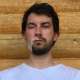# How to remove text region in a document image?

How to remove text region in a document image? Given an document image (i.e. newspaper), how to extract photos in it or remove text region?

I think traditional OCR methods may not be suitable here, as I don't need to recognize the text, and OCR is not accurate and slow. I believe text region (i.e. text blocks) and image region should be distinguishable by some threshold based methods in image processing. Any suggestions or example codes in OpenCV will be appreciated. Thanks！

BTW, what if the background color is not white, or the background color of certain blocks are not white?

Example image:edit retag close merge delete

Sort by » oldest newest most voted

My first try would follow the classic object detection pipeline, i.e. slide over the image using a fix window size, compute features for each window and classify them in text / non-text (or if you actually want the images: image / not image) . For features HOG will probably work well. If you want to detect the images then something with color will probably work better.

You could also try to detect the text using the text detection module of OpenCV, s. http://docs.opencv.org/trunk/modules/text/doc/text.html which basically does the option from above.

Good luck!

morehaha, funny, working on this problem now :)) Try to use alghortihms for keypoint detection, I desidet to use FAST, because it's realy faster than SURF and SIFT and, with param that I choosed, working better than them. ( I'm using cv::FAST(img, keyPointsFast, 100, true); )then you can use clasterization, after clasterization you will have 3 type of classes : words and strings , text and ather objectsafter that you can use differense in dispersion on X and Y for words, and if you make histograms of numPoints in colom you will see that for texts it have pereodic structureor, if FP not so bad for you, maybe you can try to use sliding window that cheks number of points and if it's greater then threshold than this is the text region :))

hmm, try to do this for your picture, result is not so good :(( maybe, you can try another paramsEDITED: haha, ups fogot to convert img to grayscale :) work not so good like on my examples, because photo with peoples have a lot of small detailsmorehere is my code adopted from squares.cpp. it finds appropriate quad areas and crop them.

the result images#include "opencv2/imgproc/imgproc.hpp"
#include "opencv2/highgui/highgui.hpp"
#include <iostream>

using namespace cv;
using namespace std;

// helper function:
// finds a cosine of angle between vectors
// from pt0->pt1 and from pt0->pt2
static double angle( Point pt1, Point pt2, Point pt0 )
{
double dx1 = pt1.x - pt0.x;
double dy1 = pt1.y - pt0.y;
double dx2 = pt2.x - pt0.x;
double dy2 = pt2.y - pt0.y;
return (dx1*dx2 + dy1*dy2)/sqrt((dx1*dx1 + dy1*dy1)*(dx2*dx2 + dy2*dy2) + 1e-10);
}

// returns sequence of squares detected on the image.
// the sequence is stored in the specified memory storage
static void findSquares( const Mat& image, vector<vector<Point> >& squares , bool inv=false)
{
squares.clear();

Mat gray,gray0;

vector<vector<Point> > contours;

cvtColor(image,gray0,COLOR_BGR2GRAY);

gray = gray0 >= 240;

if (inv)
gray = gray0 <= 10;

// find contours and store them all as a list
findContours(gray, contours, RETR_LIST, CHAIN_APPROX_SIMPLE);

vector<Point> approx;

// test each contour
for( size_t i = 0; i < contours.size(); i++ )
{
// approximate contour with accuracy proportional
// to the contour perimeter
approxPolyDP(Mat(contours[i]), approx, arcLength(Mat(contours[i]), true)*0.02, true);

// square contours should have 4 vertices after approximation
// relatively large area (to filter out noisy contours)
// and be convex.
// Note: absolute value of an area is used because
// area may be positive or negative - in accordance with the
// contour orientation
if( approx.size() == 4 &&
fabs(contourArea(Mat(approx))) > 500 &&
isContourConvex(Mat(approx)) )
{
double maxCosine = 0;

for( int j = 2; j < 5; j++ )
{
// find the maximum cosine of the angle between joint edges
double cosine = fabs(angle(approx[j%4], approx[j-2], approx[j-1]));
maxCosine = MAX(maxCosine, cosine);
}

// if cosines of all angles are small
// (all angles are ~90 degree) then write quandrange
// vertices to resultant sequence
if( maxCosine < 0.3 )
squares.push_back(approx);
}
}
}

int main( int argc, char** argv )
{
vector<vector<Point> > squares;

if( image.empty() )
{
cout <<  "Could not open or find the image" << endl ;
return -1;
}

findSquares(image, squares);

if (squares.empty())
findSquares(image, squares,true);

if (squares.empty())
{
cout <<  "Could not find any result" << endl ;
return -1;
}

// shows all the squares in the image
for( size_t i = 0; i < squares.size()-1; i++ )
{
Rect r=boundingRect( Mat(squares[i]));
Mat s= image(r);

stringstream temp_stream;
temp_stream << argv << " - "<< i << ".jpg";

imwrite(temp_stream.str(),s);
imshow(temp_stream.str(),s);
}
waitKey(0);
return 0;
}

more

Official site

GitHub

Wiki

Documentation